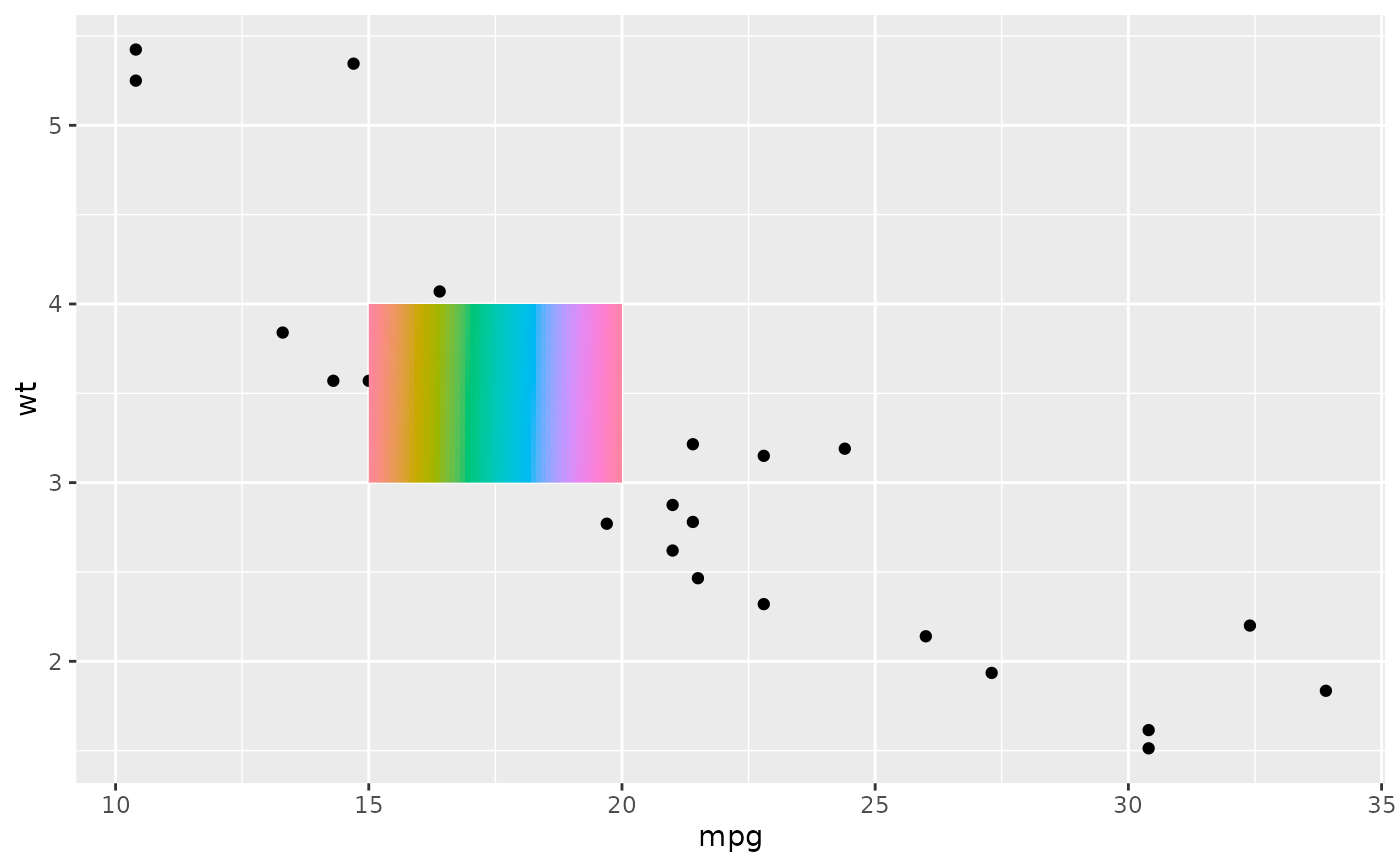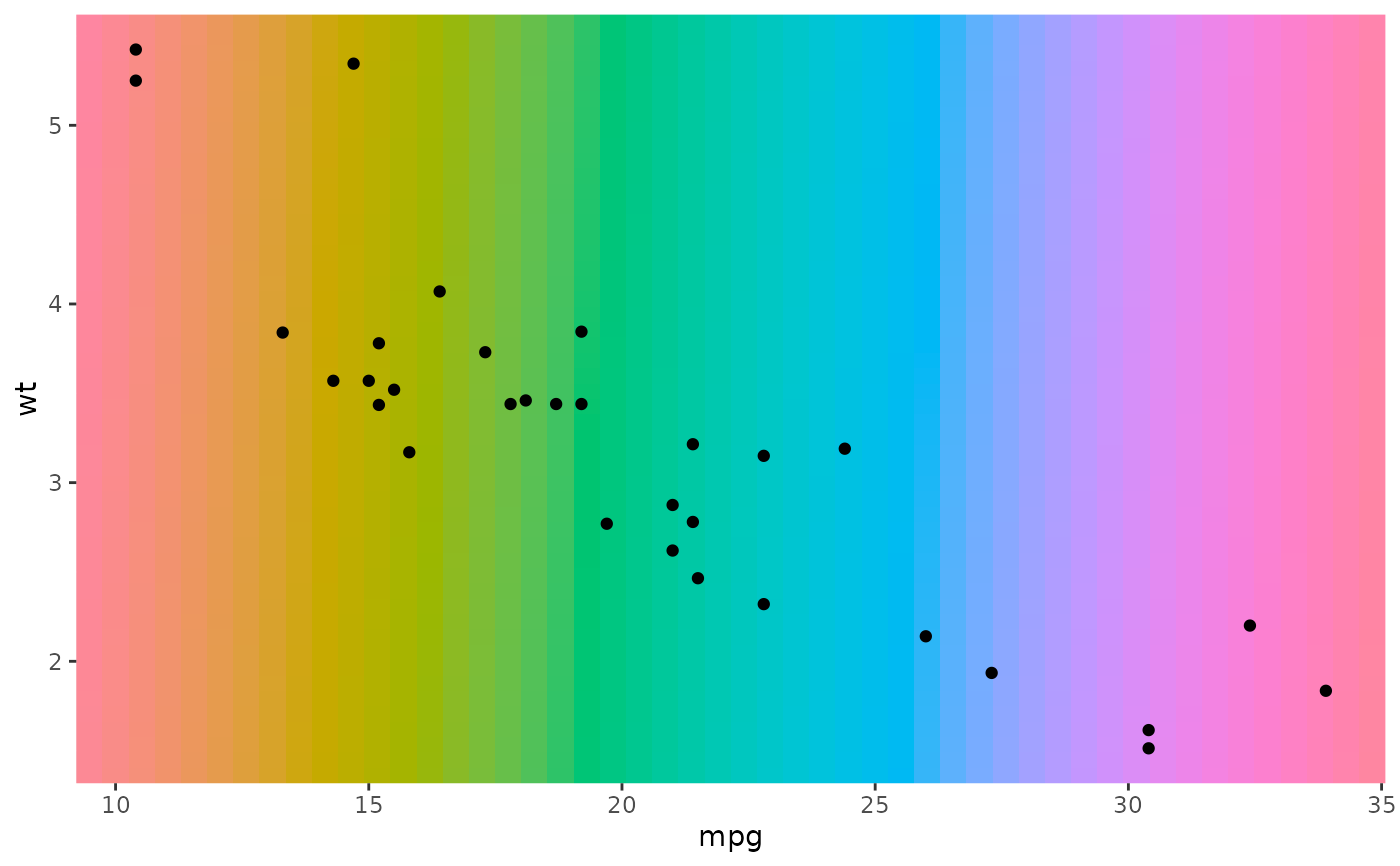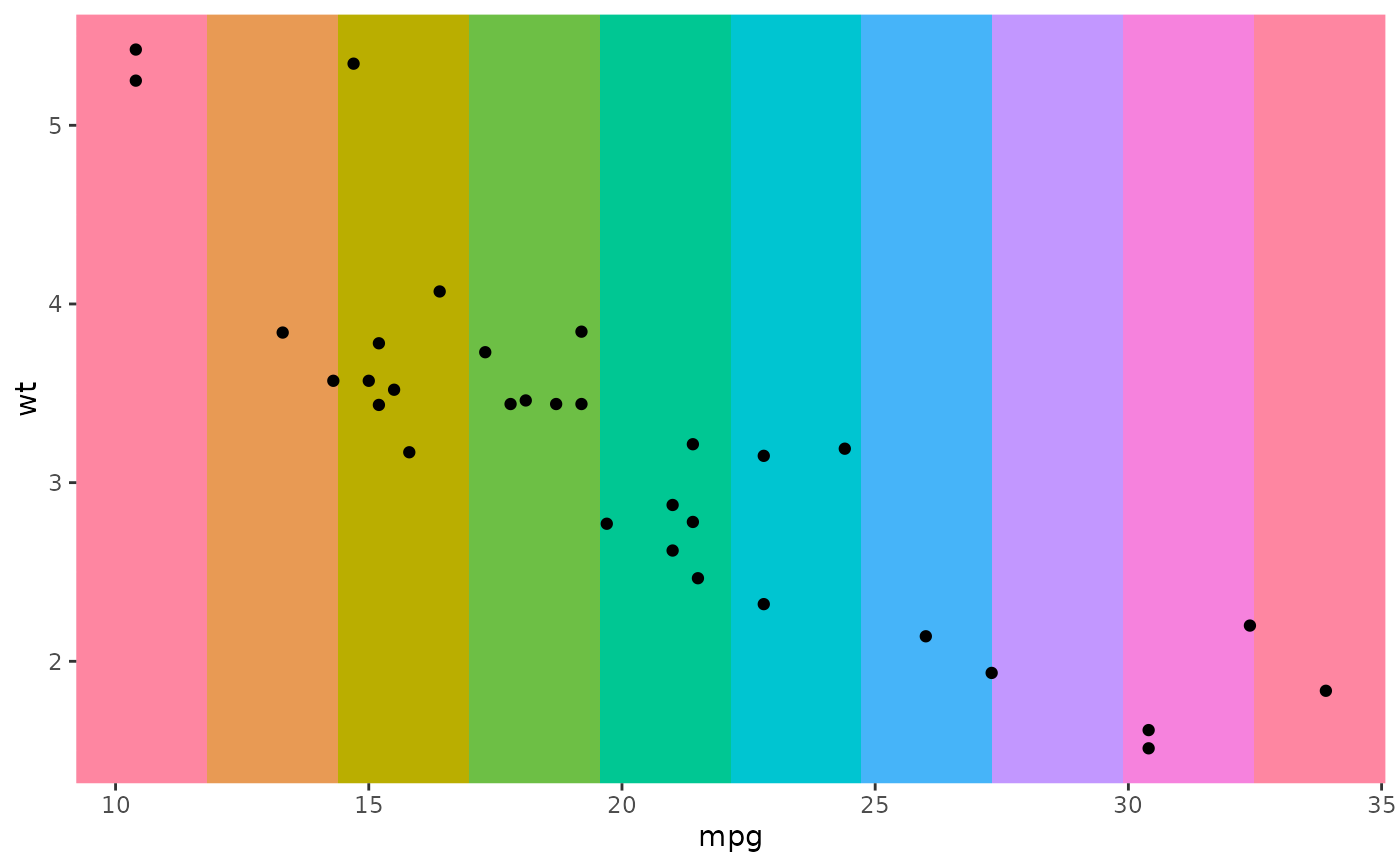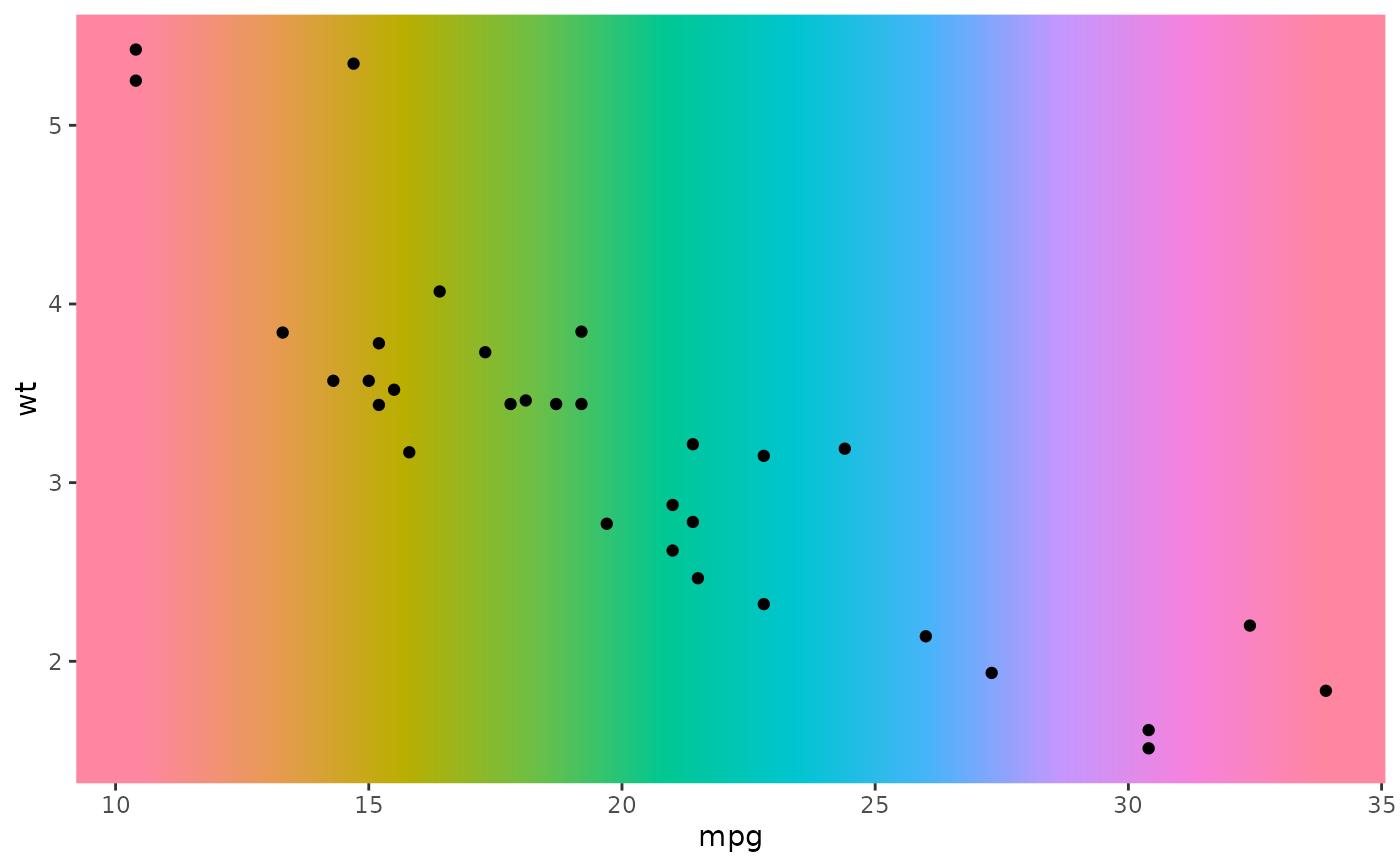This is a special version of geom_raster() optimised for static annotations that are the same in every panel. These annotations will not affect scales (i.e. the x and y axes will not grow to cover the range of the raster, and the raster must already have its own colours). This is useful for adding bitmap images.

## Usage

annotation_raster(raster, xmin, xmax, ymin, ymax, interpolate = FALSE)

## Arguments

raster

raster object to display, may be an array or a nativeRaster

xmin, xmax

x location (in data coordinates) giving horizontal location of raster

ymin, ymax

y location (in data coordinates) giving vertical location of raster

interpolate

If TRUE interpolate linearly, if FALSE (the default) don't interpolate.

## Examples

# Generate data
rainbow <- matrix(hcl(seq(0, 360, length.out = 50 * 50), 80, 70), nrow = 50)
ggplot(mtcars, aes(mpg, wt)) +
geom_point() +
annotation_raster(rainbow, 15, 20, 3, 4)# To fill up whole plot
ggplot(mtcars, aes(mpg, wt)) +
annotation_raster(rainbow, -Inf, Inf, -Inf, Inf) +
geom_point()rainbow2 <- matrix(hcl(seq(0, 360, length.out = 10), 80, 70), nrow = 1)
ggplot(mtcars, aes(mpg, wt)) +
annotation_raster(rainbow2, -Inf, Inf, -Inf, Inf) +
geom_point()rainbow2 <- matrix(hcl(seq(0, 360, length.out = 10), 80, 70), nrow = 1)
ggplot(mtcars, aes(mpg, wt)) +
annotation_raster(rainbow2, -Inf, Inf, -Inf, Inf, interpolate = TRUE) +
geom_point()